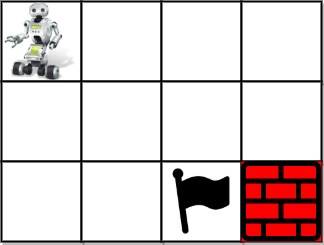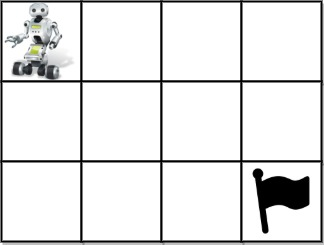## C# || Unique Paths III – How To Get The Number Of Paths From Start To End In Grid Matrix Using C#The following is a module with functions which demonstrates how to get the number of paths from start to end in a grid matrix using C#.

1. Unique Paths III – Problem Statement

You are given an m x n integer array grid where grid[i][j] could be:

• 1 representing the starting square. There is exactly one starting square.
• 2 representing the ending square. There is exactly one ending square.
• 0 representing empty squares we can walk over.
• -1 representing obstacles that we cannot walk over.

Return the number of 4-directional walks from the starting square to the ending square, that walk over every non-obstacle square exactly once.

Example 1:``` Input: grid = [[1,0,0,0],[0,0,0,0],[0,0,2,-1]] Output: 2 Explanation: We have the following two paths: 1. (0,0),(0,1),(0,2),(0,3),(1,3),(1,2),(1,1),(1,0),(2,0),(2,1),(2,2) 2. (0,0),(1,0),(2,0),(2,1),(1,1),(0,1),(0,2),(0,3),(1,3),(1,2),(2,2) ```

Example 2:``` Input: grid = [[1,0,0,0],[0,0,0,0],[0,0,0,2]] Output: 4 Explanation: We have the following four paths: 1. (0,0),(0,1),(0,2),(0,3),(1,3),(1,2),(1,1),(1,0),(2,0),(2,1),(2,2),(2,3) 2. (0,0),(0,1),(1,1),(1,0),(2,0),(2,1),(2,2),(1,2),(0,2),(0,3),(1,3),(2,3) 3. (0,0),(1,0),(2,0),(2,1),(2,2),(1,2),(1,1),(0,1),(0,2),(0,3),(1,3),(2,3) 4. (0,0),(1,0),(2,0),(2,1),(1,1),(0,1),(0,2),(0,3),(1,3),(1,2),(2,2),(2,3) ```

Example 3:``` Input: grid = [[0,1],[2,0]] Output: 0 Explanation: There is no path that walks over every empty square exactly once. Note that the starting and ending square can be anywhere in the grid. ```

2. Unique Paths III – Solution

The following is a solution which demonstrates how to get the number of paths from start to end in a grid matrix.

This solution uses Depth First Search when looking for paths.

In this solution, we find the starting coordinates and count the number of empty cells.

Then we do DFS, marking the visited positions and keeping track of the number of steps. If we reach the end and the number of steps matches the number of empty cells, we found a path.

``` 2. Unique Paths III - Solution C# // ============================================================================ // Author: Kenneth Perkins // Date: Nov 2, 2021 // Taken From: http://programmingnotes.org/ // File: Solution.cs // Description: Demonstrates how to get total paths in a grid matrix // ============================================================================ public class Solution { private int START = 1; private int END = 2; private int EMPTY = 0; private int OBSTACLE = -1; public int UniquePathsIII(int[][] grid) { // Include the starting position in the empty cell count var emptyCellCount = 1; // The row/col coordinates of the starting point var startRow = -1; var startCol = -1; for (int row = 0; row < grid.Length; ++row) { for (int col = 0; col < grid[row].Length; ++col) { // Get empty cell count if (grid[row][col] == EMPTY) { ++emptyCellCount; // Get the starting coordinates } else if (grid[row][col] == START) { startRow = row; startCol = col; } } } // Return total paths to the end return DFS(grid, startRow, startCol, emptyCellCount); } private int DFS(int[][] grid, int row, int col, int emptyCellCount) { // Make sure parameters are in bounds if (row < 0 || row >= grid.Length || col < 0 || col >= grid[row].Length) { return 0; } // Obstacle encountered or path already visited if (grid[row][col] == OBSTACLE) { return 0; } // We have reached the end if (grid[row][col] == END) { // Check to see if all empty cells have been used return emptyCellCount == 0 ? 1 : 0; } // Save the old value var oldValue = grid[row][col]; // Mark path as visited grid[row][col] = OBSTACLE; // Get the path count in right, left, top, bottom direction var pathCount = // Search Right DFS(grid, row, col + 1, emptyCellCount - 1) + // Search Left DFS(grid, row, col - 1, emptyCellCount - 1) + // Search Top DFS(grid, row - 1, col, emptyCellCount - 1) + // Search Bottom DFS(grid, row + 1, col, emptyCellCount - 1); // Unmark path as visited grid[row][col] = oldValue; return pathCount; } }// http://programmingnotes.org/ 1234567891011121314151617181920212223242526272829303132333435363738394041424344454647484950515253545556575859606162636465666768697071727374757677 // ============================================================================//    Author: Kenneth Perkins//    Date:   Nov 2, 2021//    Taken From: http://programmingnotes.org///    File:  Solution.cs//    Description: Demonstrates how to get total paths in a grid matrix// ============================================================================public class Solution {    private int START = 1;    private int END = 2;    private int EMPTY = 0;    private int OBSTACLE = -1;     public int UniquePathsIII(int[][] grid) {        // Include the starting position in the empty cell count        var emptyCellCount = 1;         // The row/col coordinates of the starting point        var startRow = -1;        var startCol = -1;         for (int row = 0; row < grid.Length; ++row) {            for (int col = 0; col < grid[row].Length; ++col) {                // Get empty cell count                if (grid[row][col] == EMPTY) {                    ++emptyCellCount;                 // Get the starting coordinates                } else if (grid[row][col] == START) {                    startRow = row;                    startCol = col;                }            }        }         // Return total paths to the end        return DFS(grid, startRow, startCol, emptyCellCount);    }     private int DFS(int[][] grid, int row, int col, int emptyCellCount) {        // Make sure parameters are in bounds        if (row < 0 || row >= grid.Length || col < 0 || col >= grid[row].Length) {            return 0;        }        // Obstacle encountered or path already visited        if (grid[row][col] == OBSTACLE) {            return 0;        }        // We have reached the end        if (grid[row][col] == END) {            // Check to see if all empty cells have been used            return emptyCellCount == 0 ? 1 : 0;        }         // Save the old value        var oldValue = grid[row][col];         // Mark path as visited        grid[row][col] = OBSTACLE;         // Get the path count in right, left, top, bottom direction        var pathCount =            // Search Right            DFS(grid, row, col + 1, emptyCellCount - 1) +            // Search Left            DFS(grid, row, col - 1, emptyCellCount - 1) +            // Search Top            DFS(grid, row - 1, col, emptyCellCount - 1) +            // Search Bottom            DFS(grid, row + 1, col, emptyCellCount - 1);         // Unmark path as visited        grid[row][col] = oldValue;         return pathCount;    }}// http://programmingnotes.org/ ```

QUICK NOTES:
The highlighted lines are sections of interest to look out for.

The code is heavily commented, so no further insight is necessary. If you have any questions, feel free to leave a comment below.

Once compiled, you should get this as your output for the example cases:

``` 2 4 0 ```